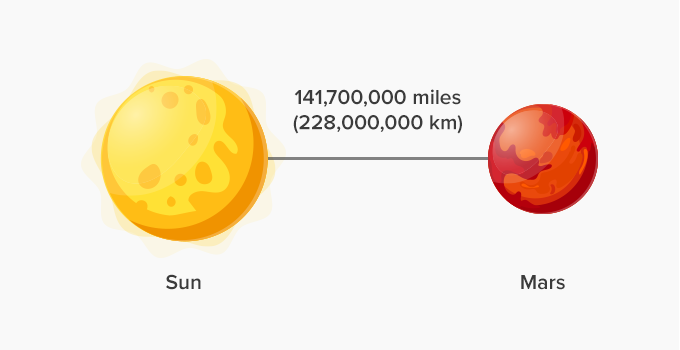# Standard Form - Definition with Examples

The Complete K-5 Math Learning Program Built for Your Child

• 40 Million Kids

Loved by kids and parent worldwide

• 50,000 Schools

Trusted by teachers across schools

• Comprehensive Curriculum

Aligned to Common Core

## What is Standard Form?

It is difficult to read numbers like 12345678900000 or 0.000000002345678. To make it easy to read very large and small numbers, we write them in standard form.

Any number that we can write as a decimal number, between 1.0 and 10.0, multiplied by a power of 10, is said to be in standard form.

1.98 ✕ 10¹³;  0.76 ✕ 10¹³ are examples of numbers in standard form.

How to write numbers in standard form:

(i) 81 900 000 000 000

• Write the first number 8

• Add a decimal point after it: 8.

• Now count the number of digits after 8. There are 13 digits.

• So, in standard form: 81 900 000 000 000 is 8.19 × 10¹³

(ii) 0.000 001 2

• Write the first non zero digit. Here it is 1

• Add a decimal point after it: 1.

• Notice that the decimal point shifts 6 places to the right.

• So, in standard form: 0.000 001 2 is 1.2 ×10ˉ⁶

Real Life Examples

Example 1: The distance between the Sun and Mars is 141,700,000 miles or 228,000,000 km.This distance can easily be written in standard form as:

1.417 × 108 miles or 2.28 × 108 km

Example 2: Atoms are tiny units of matter and are composed of three fundamental particles — proton, neutron, and electron.A proton and a neutron weigh equally, which is, 1.67 × 10–27 kg.

The weight of an electron is 9.11 × 10–31 kg

Many other quantities such as size of planets, speed of light, size of micro organisms, size of microchips, population of a country are all expressed in standard form.

Won Numerous Awards & Honors